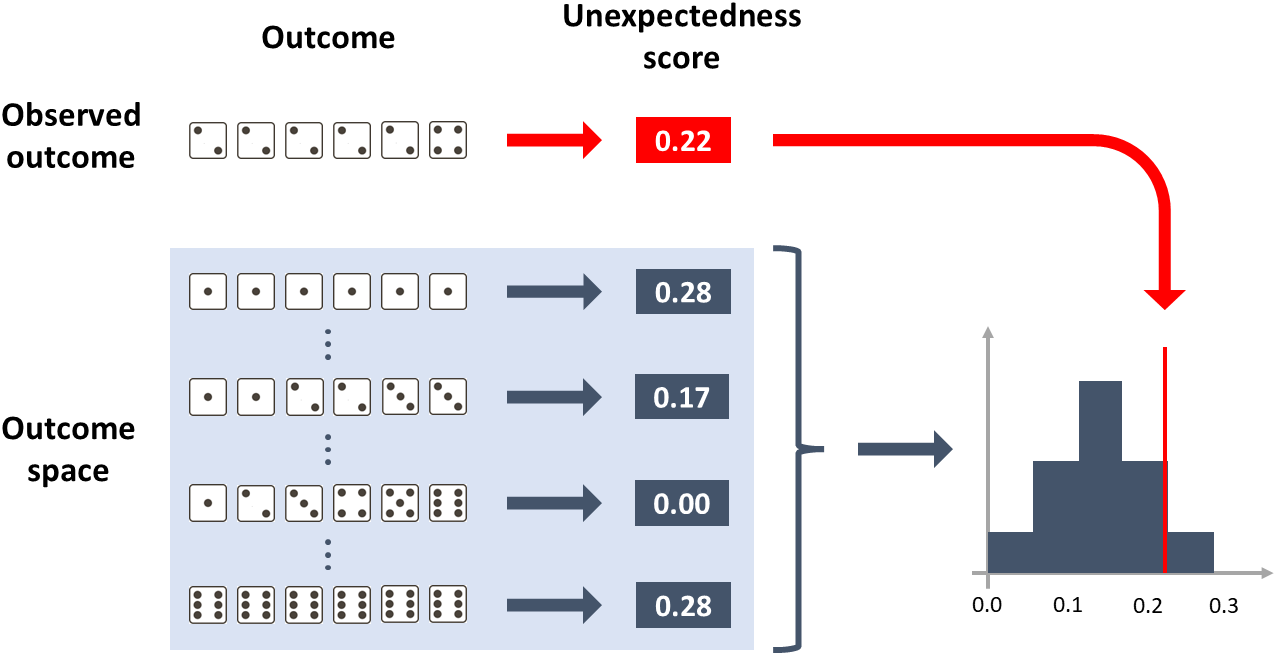# Data Scientists Need to Know Just One Statistical Test

Samuele Mazzanti

## After you read this, you will be able to test any possible statistical hypothesis. With a unique algorithm.

Asof today, Wikipedia counts a total of 104 statistical tests. As a consequence, data scientists may feel overwhelmed and ask themselves:

“Should I know all of them? And how will I know when to use one over the other?”

All the statistical tests are in reality the same one test!

Want proof? In this article, we will solve 4 very diverse statistical problems. And we will solve them always using the same exact algorithm.

1. You have thrown a die 10 times. You got [1, 1, 1, 1, 1, 2, 2, 2, 3, 3]. Is the die loaded?
2. Your friend claims that some Scrabble tiles fell out of the bag and, coincidentally, the letters formed a real word: “F-E-A-R”. You suspect that your friend is just trying make fun of you. Is your friend lying?
3. In a customer satisfaction survey, 100 customers gave an average rating of 3.00 to product A and 2.63 to product B. Is this difference significant?
4. You trained a binary classification model. It has an area under the ROC curve of 70% on your test set (made of 100 observations). Is the model significantly better than random?

Before delving into the answers to these questions, let’s try to get to the essence of what statistical testing is.

# What is the profound meaning of any statistical test?

I will try to answer this question with the least original example in statistics: the throw of a die.

Imagine you have thrown a die six times, and you got [2, 2, 2, 2, 2, 4]. A bit suspect, isn’t it? You don’t expect to get the same number 5 out of 6 times. At least, you don’t expect it to happen if the die is fair.

That’s exactly the point of statistical testing.

You have a hypothesis — called “null hypothesis” — and you want to put it to the test. Thus, you ask yourself:

“If the hypothesis was true, how often would I get an outcome as suspect as the outcome that I actually had?”

In statistics, this “how often” is called “p-value”.

Note that the concept of “unexpectedness” depends closely on the specific hypothesis that you are testing. For instance, the outcome [2, 2, 2, 2, 2, 4] is pretty weird if you think that the die is fair. However, it’s little surprising if you think that the die is loaded to get the number “2” 75% of the time.

# The ingredients of statistical testing

Reading the previous paragraph, you may have guessed that we need two ingredients:

1. The distribution of the possible outcomes, depending on the null hypothesis.
2. A measure of the “unexpectedness” of any outcome.

Regarding the 1st ingredient, it’s not always straightforward to get the full distribution of outcomes. Often, it’s more convenient (and easier) to randomly simulate a high number of outcomes: this is a good approximation of the true distribution.

About the 2nd ingredient, we need to define a function that maps each possible outcome into a single number. This number must express how unexpected the outcome is, provided that the null hypothesis is true: the more unexpected the outcome, the higher this score.

Once we have these two ingredients, the job is basically done. In fact, it’s enough to calculate the unexpectedness score of each outcome in the distribution and the unexpectedness score of the observed outcome.

The p-value is the percentage of random scores that are higher than the observed score.

That’s it. This is how every single statistical test works under the hood.

And here is a graphical representation of the process we just described:How to calculate the p-value of outcome [2, 2, 2, 2, 2, 4] under the null hypothesis of a fair die. [Image by Author]  LEGGI TUTTO https://medium.com/towards-data-science/data-scientists-need-to-know-just-one-statistical-test-3115b2ff26fd

Questo sito usa Akismet per ridurre lo spam. Scopri come i tuoi dati vengono elaborati.